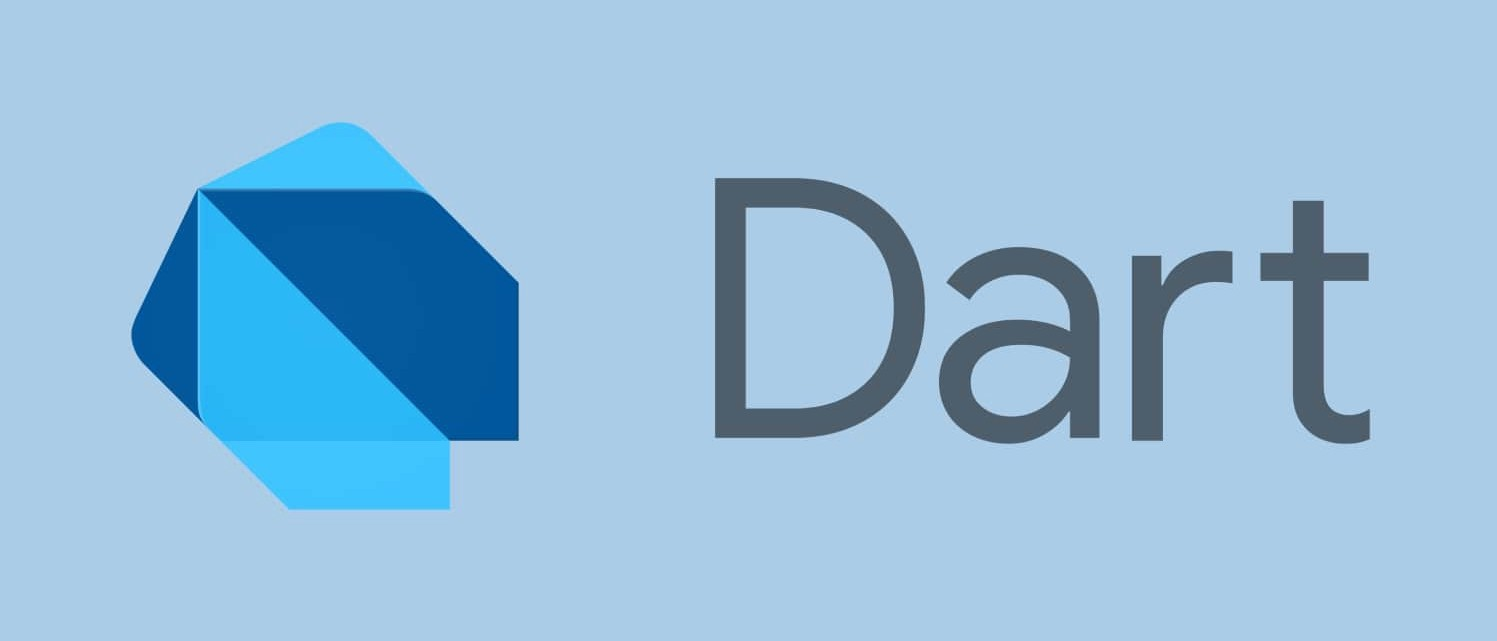## List in Dart #

List is used to store collection of data.

Let’s understand with examples

Q. How will you store name of student?

A. String Variable

Q. How will you store names 10 student?

A. 10 String Variable

We can create 10 variables but that’s very bad because we can’t create 1000 variables to store 1000 students name. Here we will List data types.

#### Example 1 #main.dart
``````void main(){

List students = ['John','Johnny', 'James'];
print(students);
}

``````

#### Index Number #

Items inside the list is known as element of that array.

Counting number starts with 1.

Index number starts with 0.

To access element of the array we have to tell the index number.

• First Element of array has index number 0.
• Second Element of array has index number 1.
• Third Element of array has index number 2.

Suppose we have a array of 200 element and to access 145the element we have to tell it’s index number which will be 144th.

#### Example 2 #main.dart
``````void main(){
List students = ['John','Johnny', 'James'];

String first_name = students;
String second_name = students;
String third_name = students;

print(first_name);
print(second_name);
print(third_name);
}

``````